3.4 LFUCache

# 最不经常最少使用 LFUCache #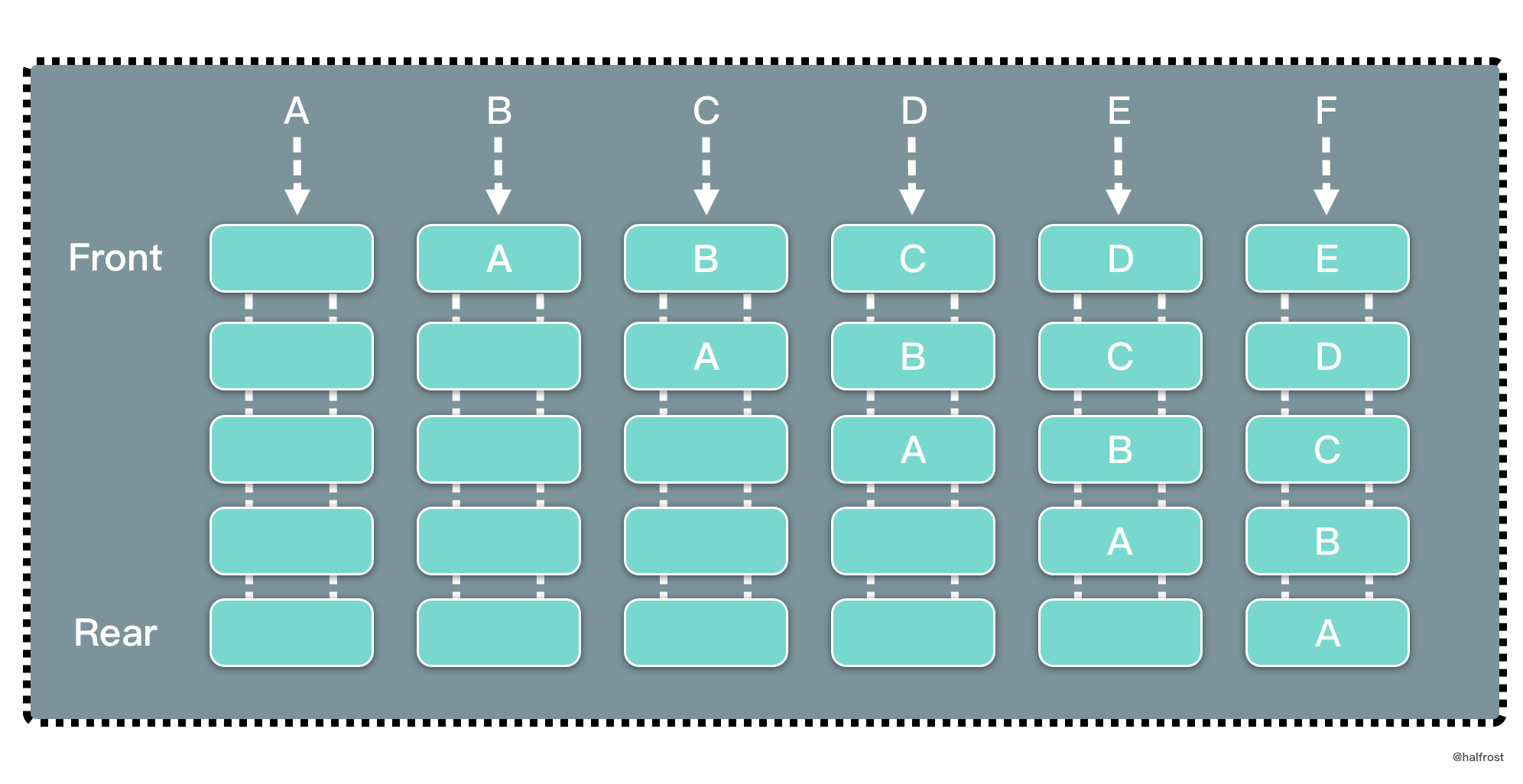LFU 是 Least Frequently Used 的缩写，即最不经常最少使用，也是一种常用的页面置换算法，选择访问计数器最小的页面予以淘汰。如下图，缓存中每个页面带一个访问计数器。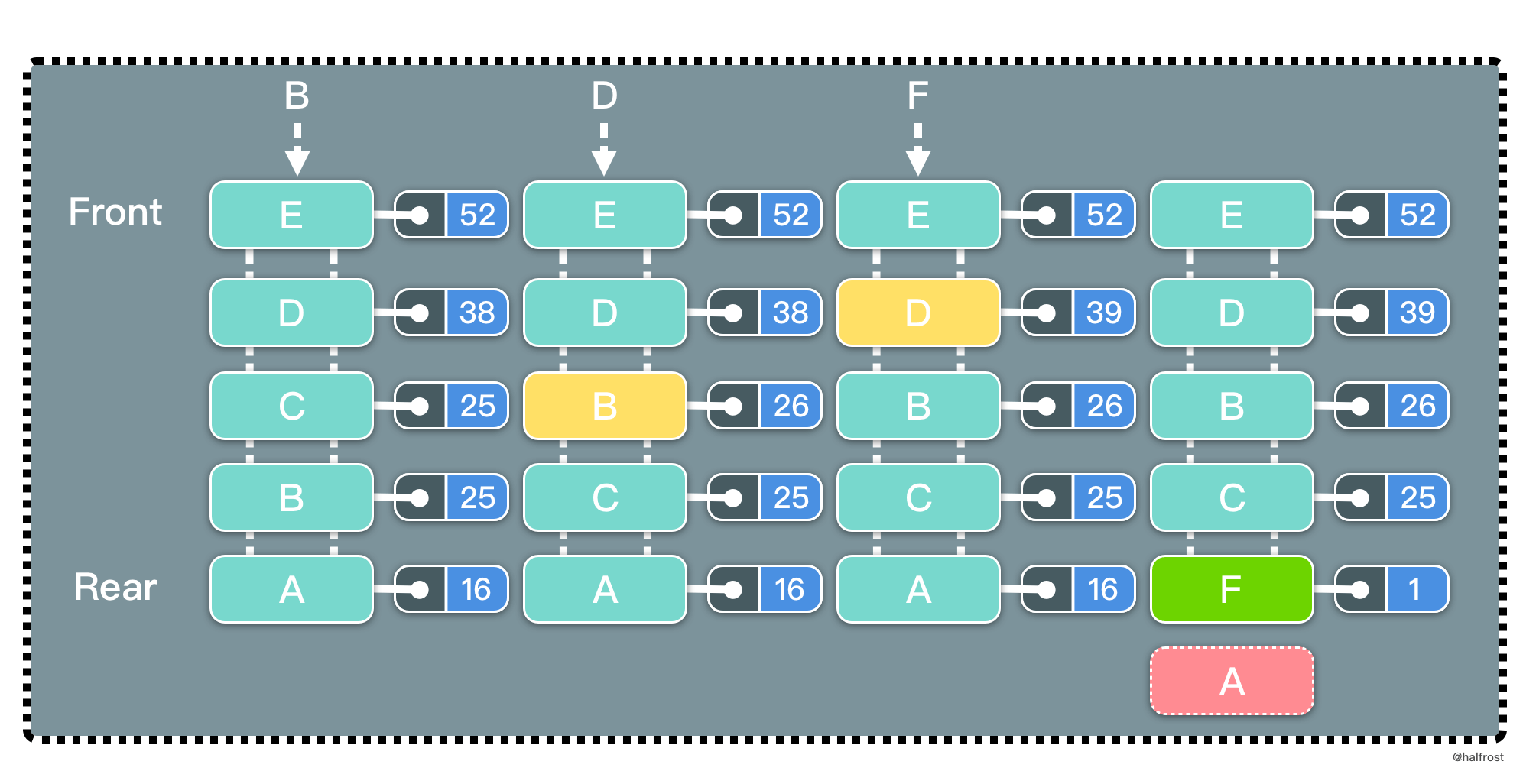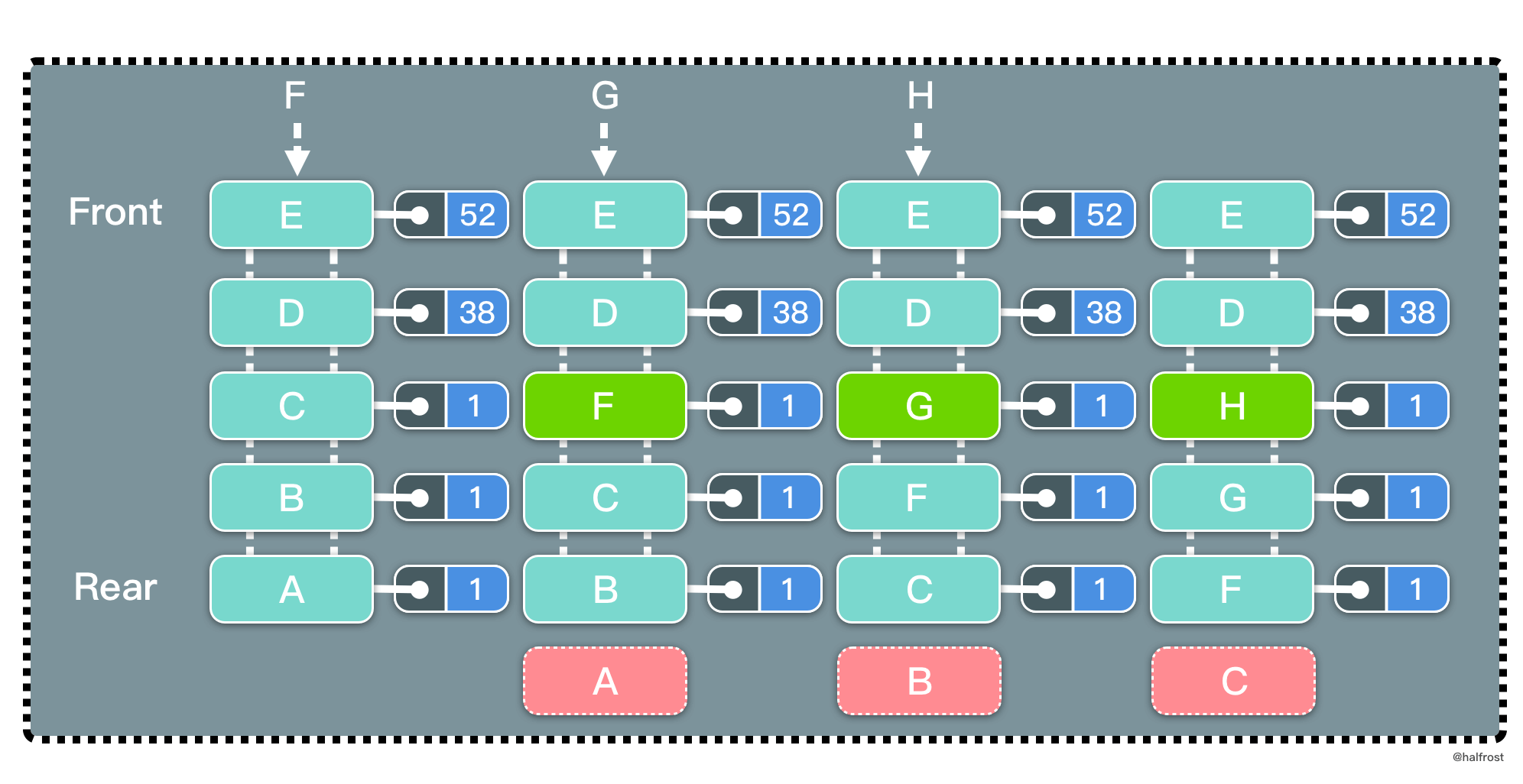## 解法一 Get O(1) / Put O(1) #

LFU 同样要求查询尽量高效，O(1) 内查询。依旧选用 map 查询。修改和删除也需要 O(1) 完成，依旧选用双向链表，继续复用 container 包中的 list 数据结构。LFU 需要记录访问次数，所以每个结点除了存储 key，value，需要再多存储 frequency 访问次数。

LFU 的更新操作和 LRU 类似，也需要用一个 map 保存 key 和双向链表结点的映射关系。这个双向链表结点中存储的是 key-value-frequency 三个元素的元组。这样通过结点中的 key 和 frequency 可以反过来删除 map 中的 key。

``````
import "container/list"

type LFUCache struct {
nodes    map[int]*list.Element
lists    map[int]*list.List
capacity int
min      int
}

type node struct {
key       int
value     int
frequency int
}

func Constructor(capacity int) LFUCache {
return LFUCache{nodes: make(map[int]*list.Element),
lists:    make(map[int]*list.List),
capacity: capacity,
min:      0,
}
}

``````

LFUCache 的 Get 操作涉及更新 frequency 值和 2 个 map。在 nodes map 中通过 key 获取到结点信息。在 lists 删除结点当前 frequency 结点。删完以后 frequency ++。新的 frequency 如果在 lists 中存在，添加到双向链表表首，如果不存在，需要新建一个双向链表并把当前结点加到表首。再更新双向链表结点作为 value 的 map。最后更新 min 值，判断老的 frequency 对应的双向链表中是否已经为空，如果空了，min++。

``````func (this *LFUCache) Get(key int) int {
value, ok := this.nodes[key]
if !ok {
return -1
}
currentNode := value.Value.(*node)
this.lists[currentNode.frequency].Remove(value)
currentNode.frequency++
if _, ok := this.lists[currentNode.frequency]; !ok {
this.lists[currentNode.frequency] = list.New()
}
newList := this.lists[currentNode.frequency]
newNode := newList.PushFront(currentNode)
this.nodes[key] = newNode
if currentNode.frequency-1 == this.min && this.lists[currentNode.frequency-1].Len() == 0 {
this.min++
}
return currentNode.value
}

``````

LFU 的 Put 操作逻辑稍微多一点。先在 nodes map 中查询 key 是否存在，如果存在，获取这个结点，更新它的 value 值，然后手动调用一次 Get 操作，因为下面的更新逻辑和 Get 操作一致。如果 map 中不存在，接下来进行插入或者删除操作。判断 capacity 是否装满，如果装满，执行删除操作。在 min 对应的双向链表中删除表尾的结点，对应的也要删除 nodes map 中的键值。

``````
func (this *LFUCache) Put(key int, value int) {
if this.capacity == 0 {
return
}
// 如果存在，更新访问次数
if currentValue, ok := this.nodes[key]; ok {
currentNode := currentValue.Value.(*node)
currentNode.value = value
this.Get(key)
return
}
// 如果不存在且缓存满了，需要删除
if this.capacity == len(this.nodes) {
currentList := this.lists[this.min]
backNode := currentList.Back()
delete(this.nodes, backNode.Value.(*node).key)
currentList.Remove(backNode)
}
// 新建结点，插入到 2 个 map 中
this.min = 1
currentNode := &node{
key:       key,
value:     value,
frequency: 1,
}
if _, ok := this.lists; !ok {
this.lists = list.New()
}
newList := this.lists
newNode := newList.PushFront(currentNode)
this.nodes[key] = newNode
}

``````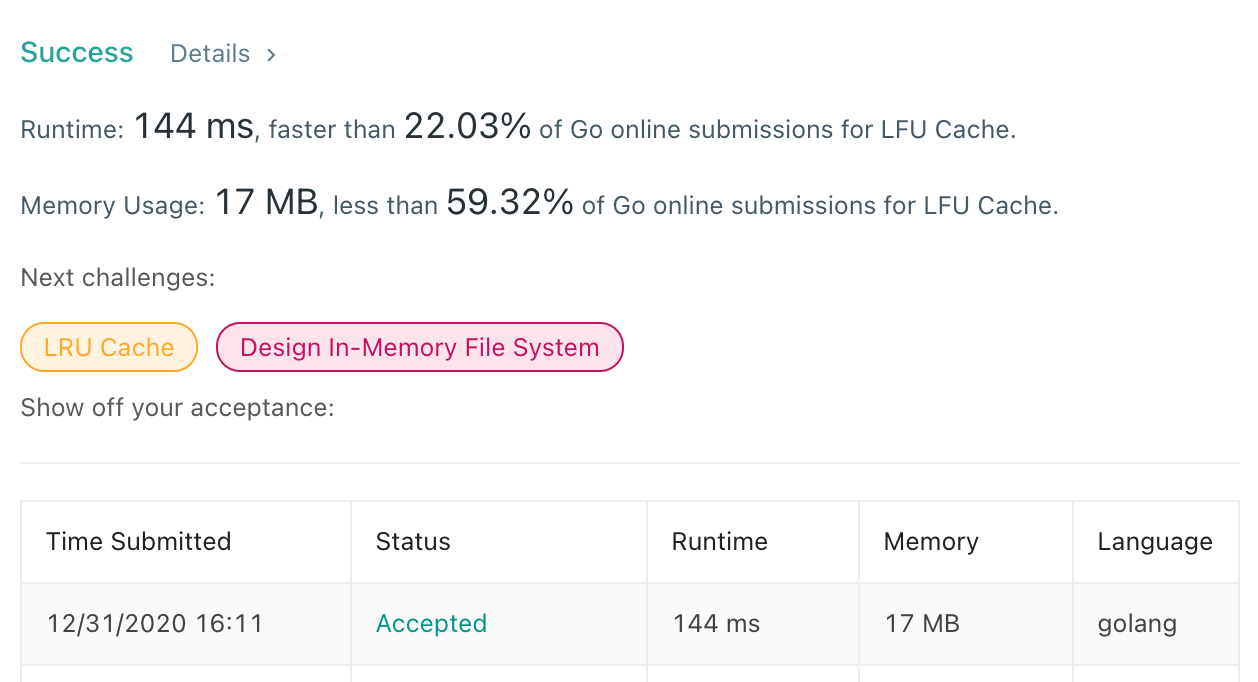## 解法二 Get O(capacity) / Put O(capacity) #

LFU 的另外一个思路是利用 Index Priority Queue 这个数据结构。别被名字吓到，Index Priority Queue = map + Priority Queue，仅此而已。

``````import "container/heap"

type LFUCache struct {
capacity int
pq       PriorityQueue
hash     map[int]*Item
counter  int
}

func Constructor(capacity int) LFUCache {
lfu := LFUCache{
pq:       PriorityQueue{},
hash:     make(map[int]*Item, capacity),
capacity: capacity,
}
return lfu
}

``````

Get 和 Put 操作要尽量的快，有 2 个问题需要解决。当访问次数相同时，如何删除掉最久的元素？当元素的访问次数发生变化时，如何快速调整堆？为了解决这 2 个问题，定义如下的数据结构：

``````// An Item is something we manage in a priority queue.
type Item struct {
value     int // The value of the item; arbitrary.
key       int
frequency int // The priority of the item in the queue.
count     int // use for evicting the oldest element
// The index is needed by update and is maintained by the heap.Interface methods.
index int // The index of the item in the heap.
}

``````

``````// A PriorityQueue implements heap.Interface and holds Items.
type PriorityQueue []*Item

func (pq PriorityQueue) Len() int { return len(pq) }

func (pq PriorityQueue) Less(i, j int) bool {
// We want Pop to give us the highest, not lowest, priority so we use greater than here.
if pq[i].frequency == pq[j].frequency {
return pq[i].count < pq[j].count
}
return pq[i].frequency < pq[j].frequency
}

func (pq PriorityQueue) Swap(i, j int) {
pq[i], pq[j] = pq[j], pq[i]
pq[i].index = i
pq[j].index = j
}

func (pq *PriorityQueue) Push(x interface{}) {
n := len(*pq)
item := x.(*Item)
item.index = n
*pq = append(*pq, item)
}

func (pq *PriorityQueue) Pop() interface{} {
old := *pq
n := len(old)
item := old[n-1]
old[n-1] = nil  // avoid memory leak
item.index = -1 // for safety
*pq = old[0 : n-1]
return item
}

// update modifies the priority and value of an Item in the queue.
func (pq *PriorityQueue) update(item *Item, value int, frequency int, count int) {
item.value = value
item.count = count
item.frequency = frequency
heap.Fix(pq, item.index)
}
``````

``````func (this *LFUCache) Get(key int) int {
if this.capacity == 0 {
return -1
}
if item, ok := this.hash[key]; ok {
this.counter++
this.pq.update(item, item.value, item.frequency+1, this.counter)
return item.value
}
return -1
}

``````

``````func (this *LFUCache) Put(key int, value int) {
if this.capacity == 0 {
return
}
this.counter++
// 如果存在，增加 frequency，再调整堆
if item, ok := this.hash[key]; ok {
this.pq.update(item, value, item.frequency+1, this.counter)
return
}
// 如果不存在且缓存满了，需要删除。在 hashmap 和 pq 中删除。
if len(this.pq) == this.capacity {
item := heap.Pop(&this.pq).(*Item)
delete(this.hash, item.key)
}
// 新建结点，在 hashmap 和 pq 中添加。
item := &Item{
value: value,
key:   key,
count: this.counter,
}
heap.Push(&this.pq, item)
this.hash[key] = item
}
``````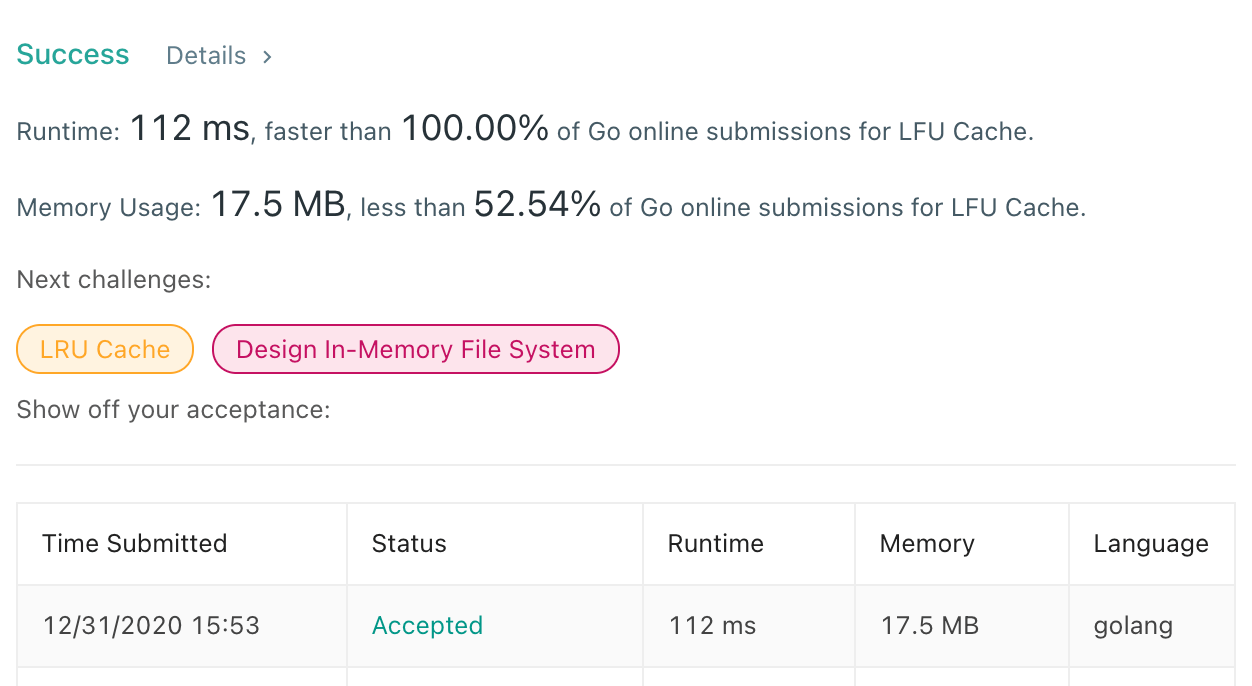## 模板 #

``````import "container/list"

type LFUCache struct {
nodes    map[int]*list.Element
lists    map[int]*list.List
capacity int
min      int
}

type node struct {
key       int
value     int
frequency int
}

func Constructor(capacity int) LFUCache {
return LFUCache{nodes: make(map[int]*list.Element),
lists:    make(map[int]*list.List),
capacity: capacity,
min:      0,
}
}

func (this *LFUCache) Get(key int) int {
value, ok := this.nodes[key]
if !ok {
return -1
}
currentNode := value.Value.(*node)
this.lists[currentNode.frequency].Remove(value)
currentNode.frequency++
if _, ok := this.lists[currentNode.frequency]; !ok {
this.lists[currentNode.frequency] = list.New()
}
newList := this.lists[currentNode.frequency]
newNode := newList.PushBack(currentNode)
this.nodes[key] = newNode
if currentNode.frequency-1 == this.min && this.lists[currentNode.frequency-1].Len() == 0 {
this.min++
}
return currentNode.value
}

func (this *LFUCache) Put(key int, value int) {
if this.capacity == 0 {
return
}
if currentValue, ok := this.nodes[key]; ok {
currentNode := currentValue.Value.(*node)
currentNode.value = value
this.Get(key)
return
}
if this.capacity == len(this.nodes) {
currentList := this.lists[this.min]
frontNode := currentList.Front()
delete(this.nodes, frontNode.Value.(*node).key)
currentList.Remove(frontNode)
}
this.min = 1
currentNode := &node{
key:       key,
value:     value,
frequency: 1,
}
if _, ok := this.lists; !ok {
this.lists = list.New()
}
newList := this.lists
newNode := newList.PushBack(currentNode)
this.nodes[key] = newNode
}

``````Nov 25, 2022Edit this page